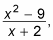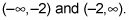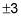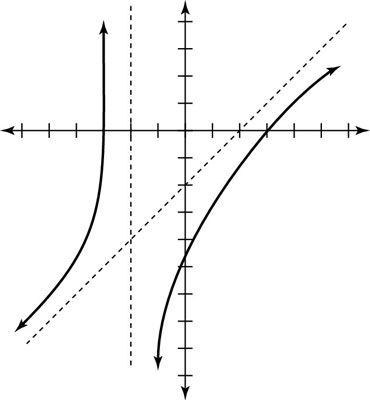##### Trigonometry Workbook For DummiesAfter you calculate all the asymptotes and the x- and y-intercepts for a rational function, you have all the information you need to start graphing the function. Rational functions where the numerator has the greater degree don’t actually have horizontal asymptotes. If the degree of the numerator of a rational function is exactly one more than the degree of its denominator, it has an oblique asymptote, which you find by using long division.

For example, if you want to graph h(x), which isthen you would follow these steps:

1. Sketch the vertical asymptote(s) of h(x).

You find only one vertical asymptote for this rational function:x = –2. And because the function has only one vertical asymptote, you find only two intervals for this graph —2. Sketch the oblique asymptote of h(x).

Because the numerator of this rational function has the greater degree, the function has an oblique asymptote. Using long division, you find that the oblique asymptote follows the equation y = x – 2.

3. Plot the x- and y-intercepts for h(x).

You find that the x-intercepts areand the y-intercept is –9/2.

4. Use test values of your choice to determine whether the graph is above or below the oblique asymptote.

Notice that the intercepts conveniently give test points in each interval. You don’t need to create your own test points, but you can if you really want to. In the first interval, the test point (–3, 0), hence the graph, is located above the oblique asymptote. In the second interval, the test points (0, –9/2) and (3, 0), as well as the graph, are located under the oblique asymptote.This figure shows the complete graph of h(x).